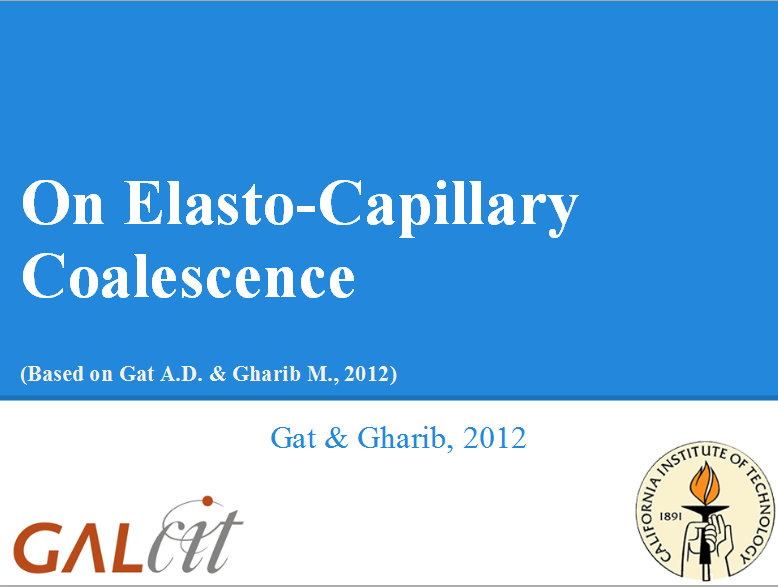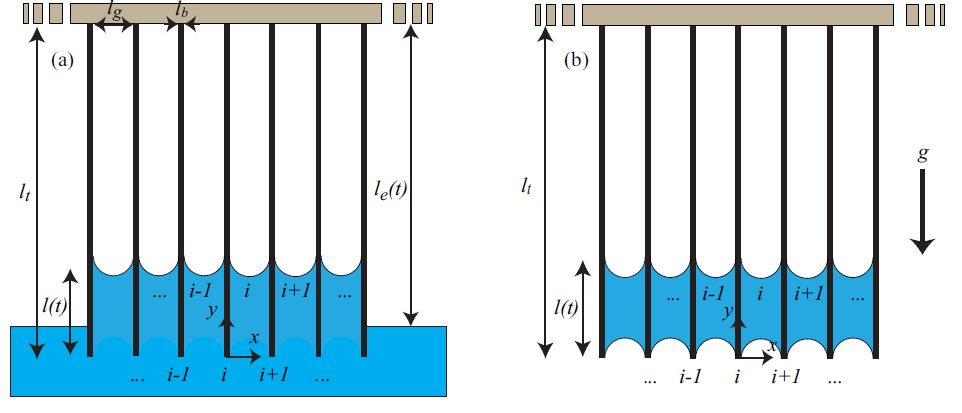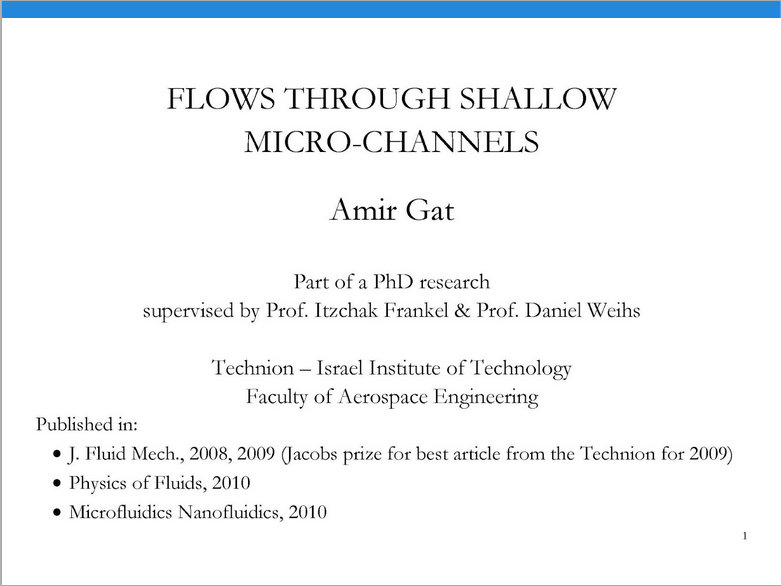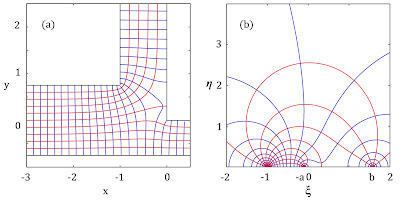# Other subjects

#### Presentation of research (click on picture to view presentation)#### Research Abstract

We analyzed two-dimensional clamped parallel elastic sheets which are partially immersed in liquid as a model for elasto-capillary coalescence. The existing literature studied this problem via minimal energy analysis of capillary and elastic energies of the post-coalescence state. Utilizing modal stability analysis and asymptotic analysis, we studied the stability of the configuration before the coalescence occurred. Our analysis revealed previously unreported relations between viscous forces, body forces, and the instability yielding the coalescence. A mathematical description of the process creating the hierarchical coalescence structure was obtained and yielded that the mean number of sheets per coalesced region is limited to the subset 2N where N is the set of natural numbers. Our results were compared with experimental data and a reasonable agreement was observed.Figure 1: Schematic description of multiple parallel elastic sheets immersed within a liquid before (a) and after (b) extraction from the liquid.

Figure 2: Length of the sheets vs. the number of collapsed sheets per coalesced region. The smooth line is the theoretical solution, the square symbols represent the experimental data and error bars mark one standard deviation. The liquid used is deionized water.
Figure 3: The influence of gravity direction on the mean number of sheets per coalesced region. In part (a) gravity is pointing toward the unclamped end of the sheets and in part (b) gravity is pointing toward the clamped end of the sheets. The liquid used is glycerol.
Figure 4: The influence of extraction speed on the number of sheets per coalesced region. In part (a) the extraction speed is 10-5m/s and in (b) the extraction speed is 5*10-3m/s. The liquid is glycerol.

#### Research Presentation (click on picture to view presentation)#### Background

Micrometer-sized systems, made possible by modern micro-fabrication techniques, enable the reduction of weight, power consumption, and production costs of various systems in the fields of heat management, flow control, fuel cells, and more. Many of these micro-systems involve gas or liquid flows through some or all of their components (Lab-on-a-Chip, micro-propulsion, gas distributors in micro-fuel cells, etc.). Microsystems play a crucial role in the modern knowledge-based economy and have a potential to create entirely new applications and significantly contribute to future science and technology. In order to achieve these goals a comprehensive understanding of the physics of flows in the micro regime is needed.

Gaseous and liquid flows through straight and uniform micro-channels have been studied extensively by numerous researchers. However, many applications involve more complex geometries and networks of micro-channels which include elements such as channel bends, constrictions and junctions. The study of gaseous and liquid flows through non-uniform configurations and micro-channel networks is therefore of considerable interest.Figure 1: Schematic description of a shallow micro-channel.

#### Compressibility and rarefaction effects

Owing to the relatively large influence of surface forces, micro-flows are typically characterized by small Reynolds numbers and nearly uniform temperatures. Furthermore, due to current manufacturing techniques, these flows often take place in the narrow gap between parallel planes. These properties suggest that Hele-Shaw model can be used to facilitate analysis of micro-fluidic applications. However, the classic Hele-Shaw approximation is limited to incompressible flows with no-slip conditions at the solid boundaries. For gaseous micro-flows, the influence of slip at the solid boundaries cannot be neglected and compressibility effects are significant even at low Mach numbers due to the dominant effect of viscous resistance. By using an auxiliary parameter, defined as a quadratic function of the pressure and the Knudsen number, the governing Navier-Stokes equations are reduced to a single Laplace equation of the auxiliary parameter. Hence, we obtained a non-trivial symmetry between compressible slip-flows and incompressible flows. This allows the use of existing analyses for incompressible no-slip flows to examine the effects of compressibility and rarefaction.

#### Modeling the influence of side wall geometry

Although the lubrication approximation yields governing equations with accuracy of the order of (H/D)(see Figure 1), the non-uniformity of the asymptotic expansion near the side walls reduced the accuracy to the order of (H/D). We developed a first-order asymptotic model which includes the influence of side wall geometry. This has required an asymptotic  solution of a singular inner region (near the side walls), an outer region correction and asymptotic matching of the two. Comparison of the higher-order model with the classic Hele-Shaw approximation have demonstrated a reduction of the error from linear to quadratic with the ratio between the configuration depth and its width, as expected from our analysis. Several commonly used side wall geometries were examined and characterized. This expansion significantly increases the usefulness of the asymptotic solutions and enable the analysis of geometries with depth to width ratios of up to 1/3.

#### Formulation of a closed-form analytic solution for flow in shallow micro-channel with general planform geometry

Using analytic function theory and the appropriate Green function a general solution for the extended asymptotic model was obtained for any planform geometry defined by a conformal mapping (Gat et al. 2009). This general analytic solution was applied for the analysis of gaseous and liquid flows through constrictions, bends and junctions (Gat et al. 2008, 2010a,b). Using the concept of ‘equivalent length’ the general solution is simplified so as to obtain explicit expressions, composed of elementary functions, which describe the viscous resistance of a given configuration as a function of geometric parameters.Figure 2: Leading order streamlines (red) and isobars (blue) of compressible weakly-rarefied flow through a shallow T-junction geometry (a) and an auxiliary plane (b).
Figure 3: Various geometries to which explicit solutions of the flow-field were obtained.

#### Application to complex micro-channel networks

The linearity of the asymptotic model enables the calculation of the viscous resistance of complex micro-channel networks by adding up the contributions of simple elements comprising the configurations.  Utilizing the computed viscous resistances of micro-channel bends and junctions, the flows through tree-like networks and parallel-channel networks are analyzed and optimized. Significant improvements of the optimization objectives (e.g. ≈ 20% reduction of viscous resistance) are achieved by relatively small modifications to the networks geometries. The accuracy of the results was validated by comparisons to numerical simulations and existing experimental data. Our model gives quantitative solutions for realistic geometries with depth to width ratio of up to ≈ 1/3 and Reynolds numbers of up to ≈ 5. The analytic method is general and can readily be applied to the design and optimization of a variety of other shallow geometries and to enable analysis and optimization of complex channel networks, which are essential components in many micro-fluidic devices.

Figure 4: A parallel channel network modelled as a combination of T-junctions (marked in gray) and optimized to create uniform mass-fluxes in the vertical channels.
Figure 5: Tree-like network (a) modelled as a combination of sharped-edged turns (b) and optimized to maximal mass-flux.